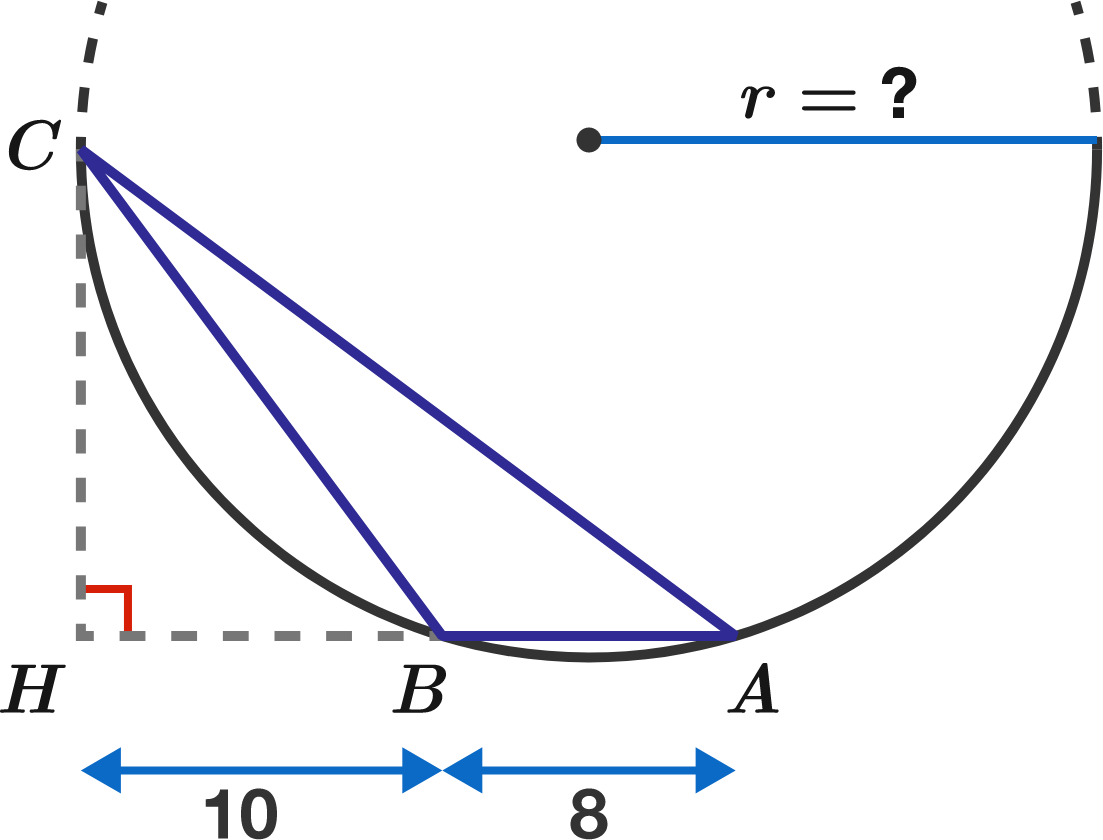# Inscribe triangle

Geometry Level 1Triangle $ABC$ is inscribed in a circle. The angle $ABC$ is obtuse, and the altitude $CH$ is tangent to the circle.

If $HB=10$ and $BA=8,$ what is the radius of the circle?

×

Problem Loading...

Note Loading...

Set Loading...